Win up to 100% scholarship on Aakash BYJU'S JEE/NEET courses with ABNAT Win up to 100% scholarship on Aakash BYJU'S JEE/NEET courses with ABNAT

# JEE Advanced Question Paper 2022 Maths Paper 1

## JEE Advanced 2022 Mathematics Question Paper 1 with Solutions

SECTION – 1 (Maximum marks : 24)

∙ This section contains EIGHT (08) questions.

∙ The answer to each question is a NUMERICAL VALUE.

∙ For each question, enter the correct numerical value of the answer using the mouse and the on-screen virtual numeric keypad in the place designated to enter the answer. If the numerical value has more than two decimal places, truncate/round-off the value to TWO decimal places.

∙ Answer to each question will be evaluated according to the following marking scheme:

Full Marks : +3 ONLY if the correct numerical value is entered;

Zero Marks : 0 In all other cases.

1. Considering only the principal values of the inverse trigonometric functions, the value of

$$\begin{array}{l}\frac{3}{2}{\cos ^{ – 1}}\sqrt {\frac{2}{{2 + {\pi ^2}}}} + \frac{1}{4}{\sin ^{ – 1}}\frac{{2\sqrt 2 \pi }}{{2 + {\pi ^2}}} + {\tan ^{ – 1}}\frac{{\sqrt 2 }}{\pi } \end{array}$$

is ______.

Sol.

$$\begin{array}{l}\frac{3}{2}{\tan ^{ – 1}}\frac{\pi }{{\sqrt 2 }} + \frac{1}{4}{\tan ^{ – 1}}\left( {\frac{{2\sqrt 2 \pi }}{{{\pi ^2} – 2}}} \right) + {\tan ^{ – 1}}\frac{{\sqrt 2 }}{\pi } \end{array}$$
$$\begin{array}{l} = \frac{\pi }{2} + \frac{1}{2}{\tan ^{ – 1}}\frac{\pi }{{\sqrt 2 }} – \frac{1}{4}{\tan ^{ – 1}}\left( {\frac{{2\sqrt 2 \pi }}{{2 – {\pi ^2}}}} \right) \end{array}$$
$$\begin{array}{l} = \frac{\pi}{2}+\frac{1}{2}\tan^{- 1}\left(\frac{\pi}{{\sqrt 2 }}\right)-\frac{1}{4}\tan^{-1}\left ( \frac{2\cdot\left ( \frac{\pi}{\sqrt2} \right )}{1-\left ( \frac{\pi}{\sqrt2} \right )^2} \right )\end{array}$$
$$\begin{array}{l} = \frac{\pi}{2} + \frac{1}{2}{\tan^{- 1}}\left( {\frac{\pi }{{\sqrt 2 }}} \right) – \frac{1}{4}\left({-\pi+2{{\tan }^{- 1}}\left( {\frac{\pi }{{\sqrt 2 }}} \right)} \right)\end{array}$$
$$\begin{array}{l} = \frac{\pi }{2} + \frac{\pi }{4} = \frac{{3\pi }}{4} \end{array}$$
$$\begin{array}{l} \simeq 2.36 \end{array}$$

2. Let α be a positive real number. Let f : and g : (α, ∞) be the functions defined by

$$\begin{array}{l}f(x) = \sin \left( {\frac{{\pi x}}{{12}}} \right)\ \text{and}\ g(x) = \frac{{2{{\log }_e}(\sqrt x – \sqrt \alpha )}}{{{{\log }_e}({e^{\sqrt x }} – {e^{\sqrt \alpha }})}}.\end{array}$$
Then the value of
$$\begin{array}{l}\displaystyle \lim_{x \to \alpha^+} f(g(x))\end{array}$$
is ______.

Sol.

$$\begin{array}{l}\displaystyle \lim_{x\to \alpha^+}g(x)=\displaystyle \lim_{x \to \alpha^+}\frac{\frac{2}{\sqrt{x}-\sqrt{\alpha}}\left ( \frac{1}{2\sqrt{x}} \right )}{\frac{1}{e^{\sqrt{x}}-e^{\sqrt{\alpha}}}\left (\frac{1}{2\sqrt{x}}\cdot e^{\sqrt{x}} \right )}\end{array}$$
$$\begin{array}{l} =\displaystyle \lim_{x\to \alpha^+}\frac{e^{\sqrt{x}}-e^{\sqrt{\alpha}}}{\sqrt{x}-\sqrt{\alpha}}\cdot \frac{1}{e^{\sqrt{x}}}\cdot 2 \end{array}$$
$$\begin{array}{l}\displaystyle \lim_{x \to {\alpha^+ }} \frac{{{e^{\sqrt x }}\cdot\,\frac{1}{{2\sqrt x }}}}{{\frac{1}{{2\sqrt x }}}}\cdot\frac{2}{{{e^{\sqrt x }}}} = 2\end{array}$$
$$\begin{array}{l}\displaystyle \lim_{x \to {\alpha ^ + }} f(g(x)) = f\left( {\mathop {\lim }\limits_{x \to {\alpha ^ + }} g(x)} \right) = \sin \frac{\pi }{6} = \frac{1}{2} = 00.50\end{array}$$

3. In a study about a pandemic, data of 900 persons was collected. It was found that

190 persons had symptom of fever,

220 persons had symptom of cough,

220 persons had symptom of breathing problem,

330 persons had symptom of fever or cough or both,

350 persons had symptom of cough or breathing problem or both,

340 persons had symptom of fever or breathing problem or both,

30 persons had all three symptoms (fever, cough and breathing problem).

If a person is chosen randomly from these 900 persons, then the probability that the person has at most one symptom is _____________.

Sol. We denote the set of people having symptoms of fever, cough and breathing problem by F, C and B respectively.

Given that n(F) = 190, n(B) = 220 and n(C) = 220

Also,

$$\begin{array}{l}n\left(F \cup C\right) = 330, n\left(C \cup B\right)=350, n\left(F \cup B\right)=340\ \text{and}\ n\left(F \cap C \cap B\right)=30\\So\ n\left(F \cap C\right) = n\left(F \right)+ n\left(C \right)-n\left(F \cup C \right)\\=80\end{array}$$

Similarly,

$$\begin{array}{l}n(F \cap B) = 70\ \text{and}\ n(C \cap B) = 90 \end{array}$$

So refer to Venn diagram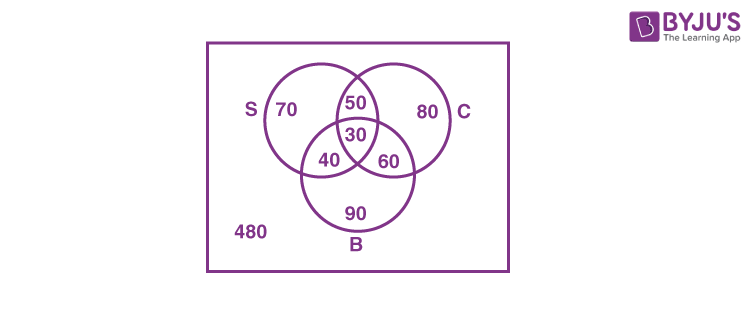Number of people having at most one symptom

= 70 + 80 + 90 + 480 = 720

$$\begin{array}{l}\text{Required probability} = \frac{{720}}{{900}} = 0.8\end{array}$$

4. Let z be a complex number with non-zero imaginary part. If

$$\begin{array}{l}\frac{{2 + 3z + 4{z^2}}}{{2 – 3z + 4{z^2}}}\ \text{is a real number},\end{array}$$

then the value of |z|2 is _________.

Sol. Let

$$\begin{array}{l}w = \frac{{4{z^2} + 3z + 2}}{{4{z^2} – 3z + 2}} = 1 + \frac{{6z}}{{4{z^2} – 3z + 2}} \end{array}$$
$$\begin{array}{l}\Rightarrow w = 1 + \frac{6}{{2\left( {2z + \frac{1}{z}} \right) – 3}}\end{array}$$
$$\begin{array}{l}\because w \in R ~\text{then} ~2z + \frac{1}{z} \in R \end{array}$$
$$\begin{array}{l}\Rightarrow 2z + \frac{1}{z} = 2\bar z + \frac{1}{{\bar z}}\end{array}$$
$$\begin{array}{l}\Rightarrow 2(z – \bar z) – \frac{{z – \bar z}}{{|\,z\,{|^2}}} = 0\end{array}$$
$$\begin{array}{l}\Rightarrow(z – \bar z)\left( {2 – \frac{1}{{|\,z\,{|^2}}}} \right) = 0 \end{array}$$
$$\begin{array}{l}\because z\; \ne \;\bar z \left(\text{given}\right)\end{array}$$

So

$$\begin{array}{l}|z|^2 = \frac{1}{2}\end{array}$$

5. Let

$$\begin{array}{l}\bar z\ \text{denote the complex conjugate of a complex number z and let}\ i = \sqrt{-1}.\end{array}$$
In the set of complex numbers, the number of distinct roots of the equation

$$\begin{array}{l}\bar z – {z^2} = i(\bar z + {z^2})\end{array}$$

is _________.

Sol.

$$\begin{array}{l}\text{Given}~ \bar z(1 – i) = {z^2}(1 + i) …\left(i\right)\\ \text{So} \left| {\bar z} \right|\left| {1 – i} \right| = {\left| z \right|^2}\left| {1 + i} \right| \end{array}$$
$$\begin{array}{l}\Rightarrow \left| z \right| = {\left| z \right|^2} \\ \Rightarrow |z| = 0~ or ~|z| = 1\end{array}$$

Let arg (z) = θ

So from (i) we get

$$\begin{array}{l}2n\pi-\theta-\frac{\pi}{4} = 2\theta+\frac{\pi}{4} \end{array}$$
$$\begin{array}{l}\Rightarrow \theta = \frac{1}{3}\left( {\frac{{4n – 1}}{2}} \right)\pi = \frac{{(4n – 1)\pi }}{6}\end{array}$$

So we will get 3 distinct values of θ. Hence there will be total 4 possible values of the complex number z.

6. Let l1, l2, …, l100 be consecutive terms of an arithmetic progression with common difference d1, and let w1, w2, …, w100 be consecutive terms of another arithmetic progression with common difference d2, where d1d2 = 10. For each i = 1, 2, …, 100, let Ri be a rectangle with length li, width wi and area Ai. If A51A50 = 1000, then the value of A100A90 is ____________.

Sol. For A.P. l1, l2, … l100

Let T1 = a and common difference = d1 and similarly for A.P. w1, w2, … w100

T1 = b and common difference = d2

A51A50 = l51w51l50w50

= (a + 50d1)(b + 50d2) – (a + 49d1)(b + 49d2)

= bd1 + ad2 + 99d1d2 = 1000

bd1 + ad2 = 10 …(i) (As d1d2 = 10)

A100A90 = l100w100l90w90

= (a + 99d1)(b + 99d2) – (a + 89d1)(b + 89d2)

= 99bd1 + 99ad2 + 992d1d2 – 89bd1 – 89ad2 – 892d1d2

= 10(bd1 + ad2) + 1880d1d2

= 10(10) + 18800

= 18900

7. The number of 4-digit integers in the closed interval [2022, 4482] formed by using the digits 0, 2, 3, 4, 6, 7 is ______.

Sol.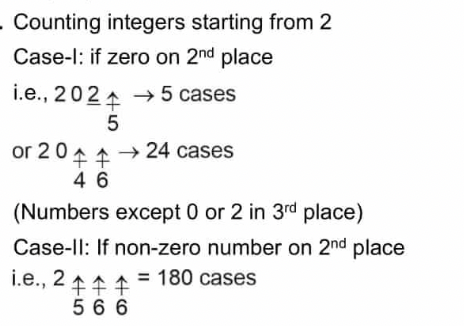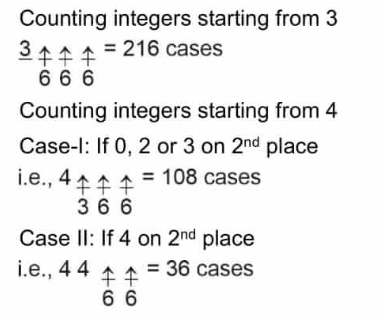∴ Total 5 + 24 + 180 + 216 + 108 + 36 = 569 numbers

8. Let ABC be the triangle with AB = 1, AC = 3 and

$$\begin{array}{l}\angle BAC = \frac{\pi }{2}. \end{array}$$
If a circle of radius r > 0 touches the sides AB, AC and also touches internally the circumcircle of the triangle ABC, then the value of r is _____________.

Sol. Let A be the origin B on the x-axis, C on the y-axis, as shown below.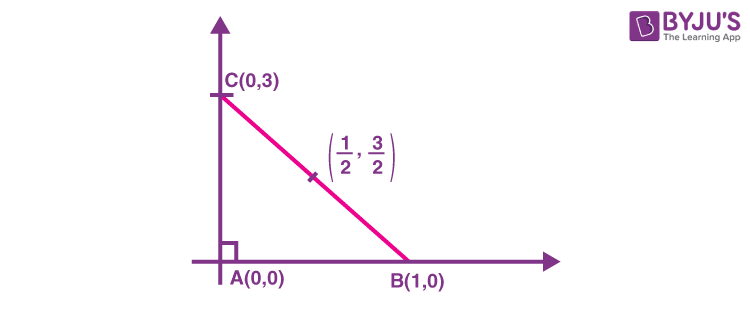∴ Equation of circumcircle is

$$\begin{array}{l}{\left( {x – \frac{1}{2}} \right)^2} + {\left( {y – \frac{3}{2}} \right)^2} = {\left( {\frac{1}{2}} \right)^2} + {\left( {\frac{3}{2}} \right)^2} = \frac{5}{2}….(1) \end{array}$$

Required circle touches AB and AC, have radius r

∴ Equation be (xr)2 + (yr)2 = r2 …(2)

If circle in equation (2) touches circumcircle internally, we have

$$\begin{array}{l}{d_{{c_1}{c_2}}} = \;|{r_1} – {r_2}| \end{array}$$
$$\begin{array}{l} \Rightarrow {\left( {\frac{1}{2} – r} \right)^2} + {\left( {\frac{3}{2} – r} \right)^2} = {\left( {\left| {\sqrt {\frac{5}{2}}- r} \right|}\right)^2}\end{array}$$
$$\begin{array}{l}\Rightarrow \frac{1}{4} + {r^2} – r + \frac{9}{4} + {r^2} – 3r = {\left( {\sqrt {\frac{5}{2}}- r} \right)^2}\;{\rm{or}}\ {\left( {r – \sqrt {\frac{5}{2}} } \right)^2}\end{array}$$
$$\begin{array}{l}\Rightarrow 2{r^2} – 4r + \frac{5}{2} = \frac{5}{2} + {r^2} – \sqrt {10} r \end{array}$$
$$\begin{array}{l}\Rightarrow r = 0\ or\ 4 – \sqrt{10} \end{array}$$

r = 0.837

= 0.84 (on rounding off)

SECTION – 2 (Maximum marks : 24)

This section contains SIX (06) questions.

Each question has FOUR options (A), (B), (C) and (D). ONE OR MORE THAN ONE of these four option(s) is(are) correct answer(s).

∙ For each question, choose the option(s) corresponding to (all) the correct answer(s).

∙ Answer to each question will be evaluated according to the following marking scheme:

Full Marks : +4 ONLY if (all) the correct option(s) is(are) chosen;

Partial Marks : +3 If all the four options are correct but ONLY three options are chosen;

Partial Marks : + 2 If three or more options are correct but ONLY two options are chosen, both of which are correct;

Partial Marks : +1 If two or more options are correct but ONLY one option is chosen and it is a correct option;

Zero Marks : 0 If none of the options is chosen (i.e. the question is unanswered);

Negative Marks : –2 In all other cases.

9. Consider the equation

$$\begin{array}{l}\int_{1}^{e}\frac{(log_e x)^{1/2}}{x(a – (log_e x)^{3/2})^2}dx,\ a\in (-\infty,0)\cup(1,\infty)\end{array}$$

Which of the following statements is/are TRUE?

(A) No a satisfies the above equation

(B) An integer a satisfies the above equation

(C) An irrational number a satisfies the above equation

(D) More than one a satisfy the above equation

Sol. Let

$$\begin{array}{l}I=\int_{1}^{e}\frac{(\ln x)^{1/2}dx}{x(a – (\ln x)^{3/2})^2}\end{array}$$

Put a – (ln x)3/2 = t

$$\begin{array}{l} \Rightarrow \;\;\; – \frac{3}{2}{(\ln x)^{1/2}} \cdot \frac{1}{x}dx = dt \end{array}$$
$$\begin{array}{l}\therefore \;\;\;I = \int\limits_a^{a – 1} {\frac{{\left( { – \frac{2}{3}} \right)dt}}{{{t^2}}}} \end{array}$$
$$\begin{array}{l}\left. { = \left( { – \frac{2}{3}} \right)\frac{{{t^{ – 2 + 1}}}}{{ – 2 + 1}}} \right|_a^{a – 1} \end{array}$$
$$\begin{array}{l}\left. { = \frac{2}{{3t}}} \right|_a^{a – 1} = \frac{2}{3}\left( {\frac{1}{{a – 1}} – \frac{1}{a}} \right) \end{array}$$
$$\begin{array}{l}\therefore I = \left( {\frac{2}{3}} \right)\frac{1}{{a(a – 1)}} = 1 \end{array}$$

⇒ 2 = 3a2 – 3a

⇒ 3a2 – 3a – 2 = 0

$$\begin{array}{l} \Rightarrow a = \frac{{3 \pm \sqrt {9 – 4(3)( – 2)} }}{6} \end{array}$$
$$\begin{array}{l}a = \frac{{3 + \sqrt {33} }}{6},\;\frac{{3 – \sqrt {33} }}{6} \end{array}$$

10. Let a1, a2, a3,… be an arithmetic progression with a1 = 7 and common difference 8. Let T1, T2, T3, … be such that T1 = 3 and Tn +1Tn = an fo n ≥ 1. Then, which of the following is/are TRUE ?

(A) T20 = 1604

(B)

$$\begin{array}{l}\sum_{k = 1}^{20} T_k=10510 \end{array}$$

(C) T30 = 3454

(D)

$$\begin{array}{l}\sum_{k = 1}^{30} T_k= 35610 \end{array}$$

Sol. Here an = 7 + (n – 1) 8 and T1 = 3

Also Tn + 1 = Tn + an

Tn = Tn–1 + an – 1

$$\begin{array}{l} \vdots \end{array}$$

T2 = T1 + a1

Tn + 1 = (Tn – 1 + an – 1) + an

= Tn – 2 + an – 2 + an – 1 + an

$$\begin{array}{l} \vdots \end{array}$$

Tn + 1 = T1 + a1 + a2 + …. + an

$$\begin{array}{l}\Rightarrow {T_{n\, + \,1}} = {T_1} + \frac{n}{2}\left[ {2\left( 7 \right) + \left( {n – 1} \right)8} \right] \end{array}$$

Tn + 1 = T1 + n(4n + 3)….(1)

∴ For n = 19 T20 = 3 + (19) (79) = 1504

For n = 29 T30 = 3 + (29) (119) = 3454 → (C)

$$\begin{array}{l}\sum\limits_{k = 1}^{20}T_k=3+\sum\limits_{k = 2}^{20}T_k=3 + \sum\limits_{k = 1}^{19} {\left( {3 + 4{n^2} + 3n} \right)}\end{array}$$
$$\begin{array}{l} = 3 + 3\left( {19} \right) + \frac{{3\left( {19} \right)\left( {20} \right)}}{2} + \frac{{4\left( {19} \right)\left( {20} \right)\left( {39} \right)}}{6} \end{array}$$

= 3 + 10507 = 10510 → (B)

And Similarly

$$\begin{array}{l}\sum_{k = 1}^{30}T_k=3 + \sum_{k = 1}^{29}{\left( {4{n^2} + 3n + 3}\right)}= 35615 \end{array}$$

11. Let P1 and P2 be two planes given by

P1: 10x + 15y + 12z – 60 = 0,

P2: –2x + 5y + 4z – 20 = 0.

Which of the following straight lines can be an edge of some tetrahedron whose two faces lie on P1 and P2?

$$\begin{array}{l}(A)\ \frac{{x – 1}}{0} = \frac{{y – 1}}{0} = \frac{{z – 1}}{5} \end{array}$$
$$\begin{array}{l}(B)\ \frac{{x – 6}}{{ – 5}} = \frac{y}{2} = \frac{z}{3} \end{array}$$
$$\begin{array}{l}(C)\ \frac{x}{{ – 2}} = \frac{{y – 4}}{5} = \frac{z}{4} \end{array}$$
$$\begin{array}{l}(D)\ \frac{x}{1} = \frac{{y – 4}}{{ – 2}} = \frac{z}{3} \end{array}$$

Sol. Equation of pair of planes is

S: (10x + 15y + 12z – 60) (–2x + 5y + 4z – 20) = 0

We will find a general point of each line and we will solve it with S. If we get more than one value of variable λ, then the line can be the edge of given tetrahedron.

(A) Point is (1, 1, 5λ + 1)

So, (60λ – 23) (20λ – 17) = 0

$$\begin{array}{l}\lambda = \frac{23}{60}\ \text{and}\ \frac{17}{20}\end{array}$$

So, it can be the edge of tetrahedron.

(B) Point is (–5λ + 6, 2λ, 3λ)

So, (16λ) (32λ – 32) = 0

⇒ λ = 0 and 1

So, it can be the edge of tetrahedron.

(C) Point is (–2λ, 5λ + 4, 4λ)

So, (103λ) (45λ) = 0

λ = 0 only

So, it cannot be the edge of tetrahedron.

(D) Point is (λ, –2λ + 4, 3λ)

⇒ (16λ) (0) = 0

It is an identity in λ, so infinitely many solutions.

Hence, it can be the edge of tetrahedron.

12. Let S be the reflection of a point Q with respect to the plane given by

$$\begin{array}{l}\overrightarrow r=-(t + p)\hat i + t\hat j + (1 + p)\hat k\end{array}$$

$$\begin{array}{l}\text{where t, p are real parameters and }\ \hat{i},\hat{j},\hat{k}\end{array}$$
are the unit vectors along the three positive coordinate axes. If the position vectors of Q and S are
$$\begin{array}{l}10\hat i + 15\hat j + 20\hat k\ \text{and}\ \alpha \hat i + \beta \hat j + \gamma \hat k \end{array}$$
respectively, then which of the following is/are TRUE?

(A) 3(α + β) = –101

(B) 3(β + γ) = –71

(C) 3(γ + α) = –86

(D) 3(α + β + γ) = –121

Sol. Equation of plane is

$$\begin{array}{l}\overrightarrow r=-\left( {t + p} \right)\hat i + t\hat j + \left( {1 + p} \right)\hat k\end{array}$$
$$\begin{array}{l}\overrightarrow r=\hat k + t\left( { – \hat i + \hat j} \right) + p\left( { – \hat i + \hat k} \right)\end{array}$$

Equation of plane in standard form is

$$\begin{array}{l}\begin{bmatrix}\vec{r}-\hat{k} & \hat{i}+\hat{j} & -\hat{i}+\hat{k} \\\end{bmatrix}=0\end{array}$$

x + y + z = 1 …(1)

Coordinate of Q = (10, 15, 20)

Coordinate of S = (α, β, γ)

$$\begin{array}{l}\therefore \frac{\alpha-10}{1}=\frac{\beta-15}{1}=\frac{\gamma-20}{1}=\frac{- 2\left( {10 + 15 + 20 – 1} \right)}{3}\end{array}$$
$$\begin{array}{l}\therefore \alpha-10 =\beta-15=\gamma-20=-\frac{{88}}{3}\end{array}$$
$$\begin{array}{l} \therefore \alpha=-\frac{58}{3},\beta=-\frac{43}{3},\gamma=-\frac{28}{3}\end{array}$$

∴ 3(α + β) = –101, 3(β + γ) = –71

3(γ + α) = –86 and 3 (α + β + γ) = –129

∴ Ans. A, B, C

13. Consider the parabola y2 = 4x. Let S be the focus of the parabola. A pair of tangents drawn to the parabola from the point P = (−2, 1) meet the parabola at P1 and P2. Let Q1 and Q2 be points on the lines SP1 and SP2 respectively such that PQ1 is perpendicular to SP1 and PQ2 is perpendicular to SP2. Then, which of the following is/are TRUE ?

(A) SQ1 = 2

$$\begin{array}{l}(B)\ {Q_1}{Q_2}=\frac{{3\sqrt {10} }}{5} \end{array}$$

(C) PQ1 = 3

(D) SQ2 = 1

Sol.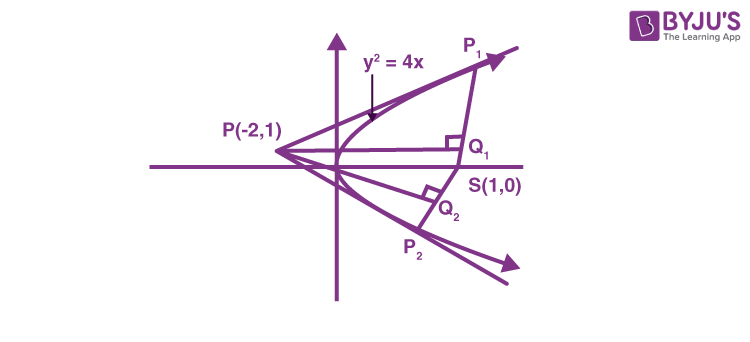Let P1(t2, 2t)then tangent at P1

ty = x + t2

Since it passes through (–2, 1)

t2t – 2 = 0

t = 2, –1

P1(4, 4) and P2(1, –2)

SP1 : 4x – 3y – 4 = 0

and SP2 : x – 1 = 0

and for Q1 :

$$\begin{array}{l}\frac{{{x_1}\; + \;2}}{4}\; = \;\frac{{{y_1}\; – \;1}}{{ – 3}}\; = \;\frac{{ – \left( { – 8\; – \;3\; – \;4} \right)}}{{25}}\; = \;\frac{3}{5} \end{array}$$
$$\begin{array}{l}\therefore {x_1}\; = \;\frac{2}{5},\;{y_1}\; = \;\frac{{ – 4}}{5} \end{array}$$

and Q2 = (1, 1)

So,

$$\begin{array}{l}SQ_1 = \sqrt {{{\left( {1\; – \;\frac{2}{5}} \right)}^2}\; + \;{{\left( {\frac{4}{5}} \right)}^2}} \; = \;1 \end{array}$$
$$\begin{array}{l}{Q_1}{Q_2}\; = \;\sqrt {\frac{9}{{25}}\; + \;\frac{{81}}{{25}}} \; = \;\sqrt {\frac{{90}}{{25}}} \; = \;\frac{{3\sqrt {10} }}{5} \end{array}$$
$$\begin{array}{l}P{Q_1}\; = \sqrt {\frac{{144}}{{25}}\; + \;\frac{{81}}{{25}}} \; = \;3 \end{array}$$

SQ2 = 1

14. Let |M| denote the determinant of a square matrix M. Let g : [0, π/2] → ℝ be the function defined by

$$\begin{array}{l}g(\theta)=\sqrt{f(\theta)-1}+\sqrt{f\left ( \frac{\pi}{2}-\theta \right )-1} \end{array}$$
where

$$\begin{array}{l}f( \theta )=\frac{1}{2}\begin{vmatrix}1 & sin\theta & 1 \\-sin\theta & 1 & sin\theta \\-1 & -sin\theta & 1 \\\end{vmatrix} + \begin{vmatrix}sin\pi & cos\left ( \theta+\frac{\pi}{4} \right ) & tan\left ( \theta-\frac{\pi}{4} \right ) \\sin\left ( \theta-\frac{\pi}{4} \right ) & -cos\frac{\pi}{2} & log_e\left ( \frac{4}{\pi} \right ) \\cot\left ( \theta+\frac{\pi}{4} \right ) & log_e\left ( \frac{\pi}{4} \right ) & tan\pi \\\end{vmatrix}\end{array}$$

Let p(x) be a quadratic polynomial whose roots are the maximum and minimum values of the function g(θ), and p(2) = 2 – √2. Then, which of the following is/are TRUE ?

(A)

$$\begin{array}{l}p\left( {\frac{{3\; + \;\sqrt 2 }}{4}} \right)\; < \;0 \end{array}$$

(B)

$$\begin{array}{l}p\left( {\frac{{1\; + \;3\sqrt 2 }}{4}} \right)\; > \;0 \end{array}$$

(C)

$$\begin{array}{l}p\left( {\frac{{5\sqrt 2 \; – \;1}}{4}} \right)\; > \;0 \end{array}$$

(D)

$$\begin{array}{l}p\left( {\frac{{5\; – \;\sqrt 2 }}{4}} \right)\; < \;0 \end{array}$$

Sol.

$$\begin{array}{l}\because f(\theta)=\frac{1}{2}\begin{vmatrix} 1 & sin\theta & 1 \\ -sin\theta & 1 & sin\theta \\ -1 & -sin\theta & 1 \\ \end{vmatrix}+\begin{vmatrix} sin\pi & cos\left ( \theta+\frac{\pi}{4} \right ) & tan\left ( \theta-\frac{\pi}{4} \right ) \\ sin\left ( \theta-\frac{\pi}{4} \right ) & -cos\frac{\pi}{2} & log_e\left ( \frac{4}{\pi} \right ) \\ cot\left ( \theta+\frac{\pi}{4} \right ) & log_e\left ( \frac{\pi}{4} \right ) & tan\pi \\ \end{vmatrix} \end{array}$$

Here

$$\begin{array}{l}\cos \;\left( {\theta \; + \;\frac{\pi }{4}} \right)\; = \; – \sin \left( {\theta \; – \;\frac{\pi }{4}} \right) \end{array}$$

and

$$\begin{array}{l}\tan \left( {\theta \; – \;\frac{\pi }{4}} \right)\; = \; – \cot \;\left( {\theta \; + \;\frac{\pi }{4}} \right) \end{array}$$

and

$$\begin{array}{l}{\log _e}\left( {\frac{4}{\pi }} \right)\; = \; – {\log _e}\;\left( {\frac{\pi }{4}} \right) \end{array}$$
$$\begin{array}{l}\text{Also sin} ~\pi = – cos \frac{\pi }{2} = tan~ \pi = 0\end{array}$$

f(θ) = 1 + sin2θ

g(θ) = |sin θ| + |cos θ|

∴ maximum and minimum values are

$$\begin{array}{l}\sqrt 2 \end{array}$$
and 1 respectively.

$$\begin{array}{l}\therefore p(x)\; = \;a\left( {x\; – \;\sqrt 2 } \right)\;\left( {x\; – \;1} \right),\end{array}$$
where aR – {0},

But p(2) = 2 – √2 then a = 1.

$$\begin{array}{l}\therefore p\left( x \right)\; = \;\left( {x\; – \;\sqrt 2 } \right)\left( {x\; – \;1} \right)\end{array}$$
$$\begin{array}{l}\therefore p\left( {\frac{{3\; + \;\sqrt 2 }}{4}} \right)\; = \;\left( {\frac{{3\; – \;3\sqrt 2 }}{4}} \right) \cdot \left( {\frac{{\sqrt 2 \; – \;1}}{4}} \right)\; < \;0\end{array}$$
$$\begin{array}{l}P\left( {\frac{{1\;\; + \;3\sqrt 2 }}{4}} \right)\; = \;\left( {\frac{{1\; – \;\sqrt 2 }}{4}} \right) \cdot \left( {\frac{{3\sqrt 2 \; – \;3}}{4}} \right)\; < \;0 \end{array}$$
$$\begin{array}{l}P\left( {\frac{{5\sqrt 2 \; – \;1}}{4}} \right)\; = \;\left( {\frac{{\sqrt 2 \; – \;1}}{4}} \right) \cdot \left( {\frac{{5\sqrt 2 \; – \;5}}{4}} \right)\; > \;0 \end{array}$$
$$\begin{array}{l}P\left( {\frac{{5\; – \;\sqrt 2 }}{4}} \right)\; = \;\left( {\frac{{5\; – \;5\sqrt 2 }}{4}} \right)\left( {\frac{{1\; – \;\sqrt 2 }}{4}} \right)\; > \;0 \end{array}$$

∴ (A) and (C) are correct.

SECTION – 3 (Maximum marks : 12)

∙ This section contains FOUR (04) Matching List Sets.

∙ Each set has ONE Multiple Choice Question.

∙ Each set has TWO lists: List-I and List-II.

List-I has Four entries (I), (II), (III) and (IV) and List-II has Five entries (P), (Q), (R), (S) and (T).

∙ FOUR options are given in each Multiple Choice Question based on List-I and List-II and ONLY ONE of these four options satisfies the condition asked in the Multiple Choice Question.

∙ Answer to each question will be evaluated according to the following marking scheme:

Full Marks : +3 ONLY if the option corresponding to the correct combination is chosen;

Zero Marks : 0 If none of the options is chosen (i.e. the question is unanswered);

Negative Marks : −1 In all other cases.

15. Consider the following lists:

 List-I List-II (I) $$\begin{array}{l}\left\{ {x\; \in \;\left[ { – \frac{{2\pi }}{3},\;\frac{{2\pi }}{3}} \right]\;:\;\cos \;x\; + \;\sin \;x\; = \;1} \right\} \end{array}$$ (P) has two elements (II) $$\begin{array}{l}\left\{ {x\; \in \;\left[ { – \frac{{5\pi }}{{18}},\;\frac{{5\pi }}{{18}}} \right]\;:\;\sqrt 3 \;\tan 3x\; = \;1} \right\} \end{array}$$ (Q) has three elements (III) $$\begin{array}{l}\left\{ {x\; \in \;\left[ { – \frac{{6\pi }}{5},\;\frac{{6\pi }}{5}} \right]\;:\;2\cos \;\left( {2x} \right)\; = \;\sqrt 3 } \right\} \end{array}$$ (R) has four elements (IV) $$\begin{array}{l}\left\{ {x\; \in \;\left[ { – \frac{{7\pi }}{4},\;\frac{{7\pi }}{4}} \right]\;:\;\sin \;x\; – \;cos\;x\; = \;1} \right\} \end{array}$$ (S) has five elements (T) has six elements

The correct option is:

(A) (I) → (P); (II) → (S); (III) → (P); (IV) → (S)

(B) (I) → (P); (II) → (P); (III) → (T); (IV) → (R)

(C) (I) → (Q); (II) → (P); (III) → (T); (IV) → (S)

(D) (I) → (Q); (II) → (S); (III) → (P); (IV) → (R)

Sol. (i)

$$\begin{array}{l}\left\{ {x \in \left[ {\frac{{ – 2\pi }}{3},\;\frac{{2\pi }}{3}} \right],\;\cos x\; + \;\sin x\; = \;1} \right\} \end{array}$$

cosx + sinx = 1

$$\begin{array}{l}\sin \left( {\frac{\pi }{4}\; + \;x} \right)\; = \;\frac{1}{{\sqrt 2 }} \end{array}$$
$$\begin{array}{l}\frac{\pi }{4}\; + \;x\; = \;n\pi \; + \;{\left( { – 1} \right)^n}\frac{\pi }{4} \end{array}$$
$$\begin{array}{l}x\; = \;n\pi \; + \;{\left( { – 1} \right)^n}\frac{\pi }{4}\; – \frac{\pi }{4} \end{array}$$

x has 2 elements. → P

(ii)

$$\begin{array}{l}\left\{ {x \in \left[ {\frac{{ – 5\pi }}{{18}},\;\frac{{5\pi }}{{18}}} \right]:\;\sqrt 3 \tan 3x\; = \;1} \right\} \end{array}$$
$$\begin{array}{l}\sqrt 3 \;\tan 3x\; = 1 \end{array}$$
$$\begin{array}{l}\tan 3x\; = \;\frac{1}{{\sqrt 3 }} \end{array}$$
$$\begin{array}{l}3x\; = \;n\pi \; + \;\frac{\pi }{6} \end{array}$$
$$\begin{array}{l}x\; = \;\frac{{n\pi }}{3}\; + \;\frac{\pi }{{18}} \end{array}$$

x has 2 elements. → P

(iii)

$$\begin{array}{l}\left\{ {x \in \left[ {\frac{{ – 6\pi }}{5},\;\frac{{6\pi }}{5}} \right]:\;2\cos 2x\; = \;\sqrt 3 } \right\} \end{array}$$
$$\begin{array}{l}2\cos 2x\; = \;\sqrt 3 \end{array}$$
$$\begin{array}{l}\cos 2x\; = \;\frac{{\sqrt 3 }}{2} \end{array}$$
$$\begin{array}{l}2x\; = \;2n\pi \; \pm \frac{\pi }{6} \end{array}$$
$$\begin{array}{l}x\; = \;n\pi \; \pm \frac{\pi }{{12}} \end{array}$$

x has 6 elements. → T

(iv)

$$\begin{array}{l}\left\{ {x \in \left[ {\frac{{ – 7\pi }}{4},\;\frac{{7\pi }}{4}} \right]:\;\sin x\; – \;\cos x\; = \;1} \right\} \end{array}$$

sinx – cosx = 1

$$\begin{array}{l}\sin \left( {x\; – \;\frac{\pi }{4}} \right)\; = \;\frac{1}{{\sqrt 2 }} \end{array}$$
$$\begin{array}{l}x\; – \;\frac{\pi }{4}\; = \;n\pi \; + \;{\left( { – 1} \right)^n}\;\frac{\pi }{4} \end{array}$$
$$\begin{array}{l}x\; = \;n\pi \; + \;{\left( { – 1} \right)^n}\frac{\pi }{4}\; + \;\frac{\pi }{4} \end{array}$$

x has 4 elements. → R

∴ option B is correct.

16. Two players, P1 and P2, play a game against each other. In every round of the game, each player rolls a fair die once, where the six faces of the die have six distinct numbers. Let x and y denote the readings on the die rolled by P1 and P2, respectively. If x > y, then P1 scores 5 points and P2 scores 0 point. If x = y, then each player scores 2 points. If x < y, then P1 scores 0 point and P2 scores 5 points. Let Xi and Yi be the total scores of P1 and P2, respectively, after playing the ith round.

 List-I List-II (I) Probability of (X2 ≥ Y2) is (P) 3/8 (II) Probability of (X2 > Y2) is (Q) 11/16 (III) Probability of (X3 = Y3) is (R) 5/16 (IV) Probability of (X3 > Y3) is (S) 355/864 (T) 77/432

The correct option is:

(A) (I) → (Q); (II) → (R); (III) → (T); (IV) → (S)

(B) (I) → (Q); (II) → (R); (III) → (T); (IV) → (T)

(C) (I) → (P); (II) → (R); (III) → (Q); (IV) → (S)

(D) (I) → (P); (II) → (R); (III) → (Q); (IV) → (T)

Sol. P(Xi > Yi) + P(Xi < Yi) + P(Xi = Yi) = 1

and P(Xi > Yi) = P(Xi < Yi) = p

for i = 2

P(X2 = Y2) = 2p(x > y). p(x < y) + (p(x = y))2

$$\begin{array}{l} = 2.\frac{{^6{C_2}}}{{36}}.\frac{{^6{C_2}}}{{36}} + {\left( {\frac{{^6{C_1}}}{{36}}} \right)^2} \end{array}$$
$$\begin{array}{l} = \frac{{25}}{{72}} + \frac{1}{{36}} = \frac{{27}}{{72}} = \frac{3}{8} \end{array}$$
$$\begin{array}{l}P({X_2}\,\, > \,\,{Y_2}) = \frac{1}{2}\left( {1 – \frac{3}{8}} \right) = \frac{5}{{16}} \end{array}$$
$$\begin{array}{l}P({X_2}\geq{Y_2}) =\frac{5}{16}+\frac{3}{8}=\frac{11}{16}\end{array}$$

I → Q, II → R

for i = 3

P(X3 = Y3) = 6. p(x > y). p(x < y) p(x = y) + (p(x = y))3

$$\begin{array}{l} = 6.\frac{{^6{C_2}}}{{36}}.\frac{{^6{C_2}}}{{36}}.\frac{{^6{C_1}}}{{36}} + {\left( {\frac{{^6{C_1}}}{{36}}} \right)^3} \end{array}$$
$$\begin{array}{l} = \frac{77}{432} \end{array}$$
$$\begin{array}{l}P({X_3}\,\, > \,\,{Y_3}) = \frac{1}{2}\left( {1 – \frac{{77}}{{432}}} \right) \end{array}$$
$$\begin{array}{l} = \frac{355}{864} \end{array}$$

III → T, IV → S

17. Let p, q, r be non-zero real numbers that are, respectively, the 10th, 100th and 1000th terms of a harmonic progression. Consider the system of linear equations

x + y + z = 1

10x + 100y + 1000z = 0

qr x + pr y + pq z = 0

 List-I List-II (I) If $$\begin{array}{l}\frac{q}{r} = 10, \end{array}$$ then the system of linear equations has (P) x = 0, $$\begin{array}{l}y = \frac{{10}}{9},\,\,z = – \frac{1}{9} \end{array}$$ as a solution (II) If $$\begin{array}{l}\frac{p}{r} \ne 100, \end{array}$$ then the system of linear equations has (Q) $$\begin{array}{l}x = \frac{{10}}{9},\,\,y = – \frac{1}{9},\,\,z = 0 \end{array}$$ as a solution (III) If $$\begin{array}{l}\frac{p}{q} \ne 10, \end{array}$$ then the system of linear equations has (R) infinitely many solutions (IV) If $$\begin{array}{l}\frac{p}{q} = 10, \end{array}$$ then the system of linear equations has (S) no solution (T) at least one solution

The correct option is:

(A) (I) → (T); (II) → (R); (III) → (S); (IV) → (T)

(B) (I) → (Q); (II) → (S); (III) → (S); (IV) → (R)

(C) (I) → (Q); (II) → (R); (III) → (P); (IV) → (R)

(D) (I) → (T); (II) → (S); (III) → (P); (IV) → (T)

Sol. x + y + z = 1 ___(1)

10x + 100y + 1000z = 0 ___(2)

qrx + pry + pqz = 0 ___(3)

Equation (3) can be re-written as

$$\begin{array}{l}\frac{x}{p} + \frac{y}{q} + \frac{z}{r} = 0 \end{array}$$
(∵ p, q, r ≠ 0)

Let

$$\begin{array}{l}p = \frac{1}{{a + 9d}},\,\,q = \frac{1}{{a + 99d}},\,\,r = \frac{1}{{a + 999d}} \end{array}$$

Now, equation (3) is

(a + 9d)x + (a + 99d)y + (a + 999d)z = 0

$$\begin{array}{l}\Delta=\begin{vmatrix}1 & 1 & 1 \\10 & 100 & 1000 \\a+9d & a+99d & a+999d \\\end{vmatrix}=0\end{array}$$
$$\begin{array}{l}\Delta_x=\begin{vmatrix}1 & 1 & 1 \\0 & 100 & 1000 \\0 & a+99d & a+999d \\\end{vmatrix}=900(d-a)\end{array}$$
$$\begin{array}{l}\Delta_y=\begin{vmatrix}1 & 1 & 1 \\10 & 0 & 1000 \\a+9d & 0 & a+999d \\\end{vmatrix}=990(a-d)\end{array}$$
$$\begin{array}{l}\\Delta_z=\begin{vmatrix}1 & 1 & 1 \\10 & 100 & 0 \\a+9d & a+99d & 0\\\end{vmatrix}=90(d-a)\end{array}$$

Option I: If q/r = 10 ⇒ a = d

Δ = Δx = Δy = Δz = 0

And eq. (1) and eq. (2) represents non-parallel planes and eq. (2) and eq. (3) represents same plane

⇒ Infinitely many solutions

I → P, Q, R, T

Option II :

$$\begin{array}{l}\frac{p}{r} \ne 100 \Rightarrow a \ne d \end{array}$$

Δ = 0, Δx, Δy, Δz ≠ 0

No solution

II → S

Option III:

$$\begin{array}{l}\frac{p}{q} \ne 10 \Rightarrow a \ne d \end{array}$$

No solution

III → S

Option IV: If

$$\begin{array}{l}\frac{p}{q} = 10 \Rightarrow a = d \end{array}$$

Infinitely many solutions

IV → P, Q, R, T

18. Consider the ellipse

$$\begin{array}{l}\frac{{{x^2}}}{4} + \frac{{{y^2}}}{3} = 1 \end{array}$$

Let H(α, 0), 0< α < 2, be a point. A straight line drawn through H parallel to the y-axis crosses the ellipse and its auxiliary circle at points E and F respectively, in the first quadrant. The tangent to the ellipse at the point E intersects the positive x-axis at a point G. Suppose the straight line joining F and the origin makes an angle φ with the positive x-axis.

 List-I List-II (I) If Φ = π/4, then the area of the triangle FGH is $$\begin{array}{l}(P)\ \frac{(\sqrt{3}-1)^4}{8}\end{array}$$ (II) If Φ = π/3, then the area of the triangle FGH is (Q) 1 (III) If Φ = π/6, then the area of the triangle FGH is (R) 3/4 (IV) If Φ = π/12, then the area of the triangle FGH is $$\begin{array}{l}(S)\ \frac{1}{2\sqrt{3}}\end{array}$$ $$\begin{array}{l}(T)\ \frac{3\sqrt{3}}{2}\end{array}$$

The correct option is:

(A) (I) → (R); (II) → (S); (III) → (Q); (IV) → (P)

(B) (I) → (R); (II) → (T); (III) → (S); (IV) → (P)

(C) (I) → (Q); (II) → (T); (III) → (S); (IV) → (P)

(D) (I) → (Q); (II) → (S); (III) → (Q); (IV) → (P)

Sol.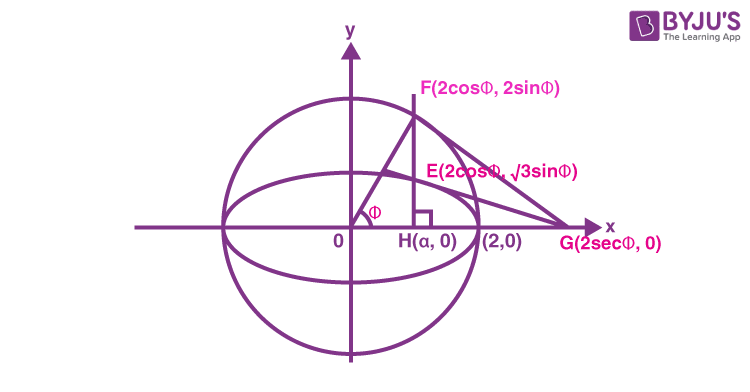$$\begin{array}{l}\alpha\equiv 2\cos \phi \end{array}$$

Tangent at

$$\begin{array}{l}E\left( {2\cos \phi ,\,\,\sqrt 3 \sin \phi } \right) \end{array}$$
to ellipse
$$\begin{array}{l}\frac{{{x^2}}}{4} + \frac{{{y^2}}}{3} = 1 \end{array}$$

i.e.

$$\begin{array}{l}\frac{{x\cos \phi }}{2} + \frac{{y\sin \phi }}{{\sqrt 3 }} = 1 \end{array}$$
intersect x-axis at
$$\begin{array}{l}G(2\sec \phi ,\,\,0) \end{array}$$
$$\begin{array}{l}\text{Area of triangle}=\frac{1}{2}\left({2\sec\phi-2\cos \phi} \right)2\sin \phi \end{array}$$
$$\begin{array}{l}\Delta= 2{\sin ^2}\phi .\,\,\tan \phi \end{array}$$
$$\begin{array}{l}\Delta=(1 – \cos 2\phi ).\tan \phi \end{array}$$

I. If

$$\begin{array}{l}\phi=\frac{\pi}{4},\ \Delta=1\to(Q) \end{array}$$

II. If

$$\begin{array}{l}\phi=\frac{\pi }{3},\ \Delta=2.{\left({\frac{{\sqrt 3 }}{2}}\right)^2}.\sqrt 3=\frac{{3\sqrt 3}}{2}\to (T) \end{array}$$

III. If

$$\begin{array}{l}\phi=\frac{\pi }{6},\ \Delta=2.{\left({\frac{1}{2}} \right)^2}.\frac{1}{{\sqrt 3 }} = \frac{1}{{2\sqrt 3 }} \to (S) \end{array}$$

IV. If

$$\begin{array}{l}\phi=\frac{\pi }{{12}},\ \Delta=\left( {1 – \frac{{\sqrt 3 }}{2}} \right).\left( {2 – \sqrt 3 } \right) = \frac{{{{\left( {2 – \sqrt 3 } \right)}^2}}}{2} \to (P) \end{array}$$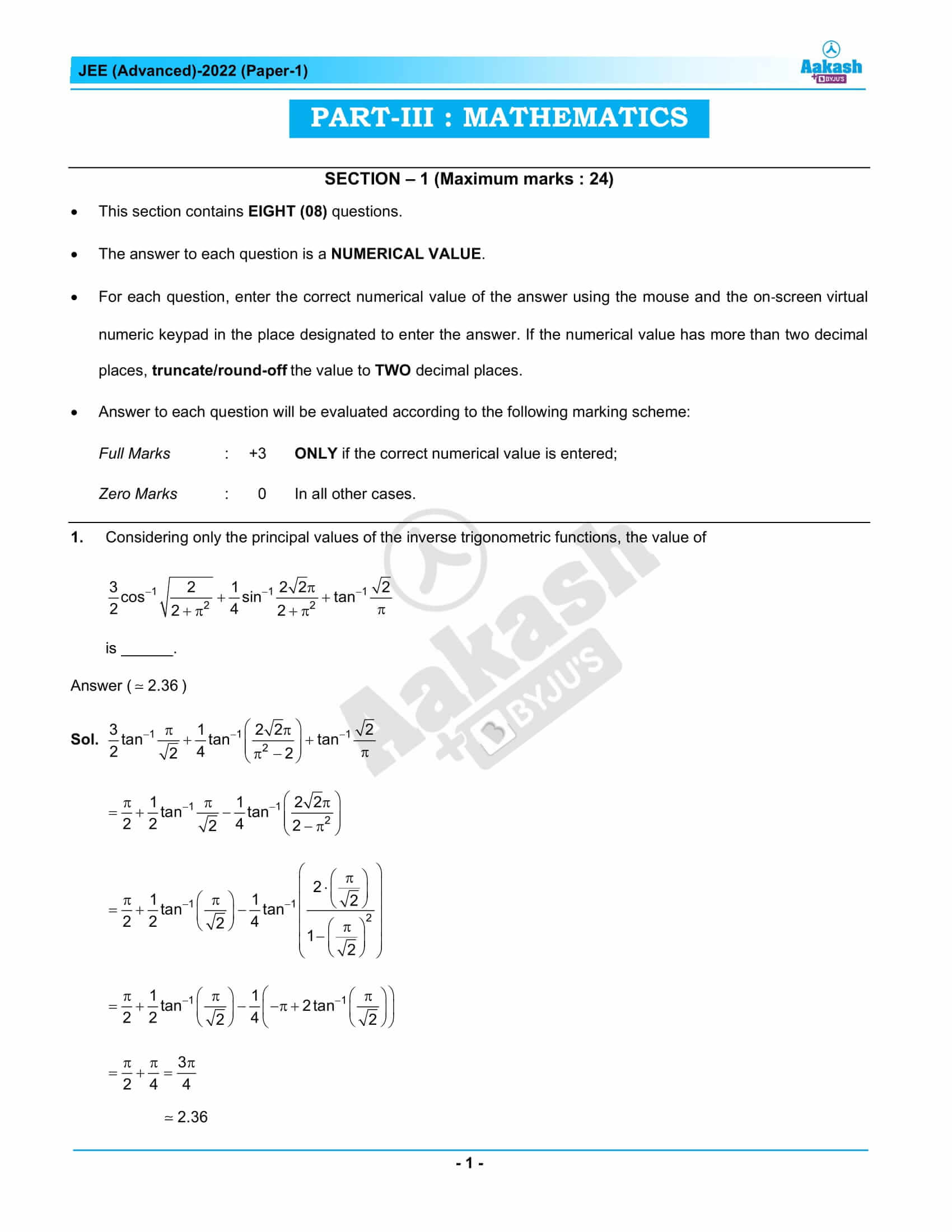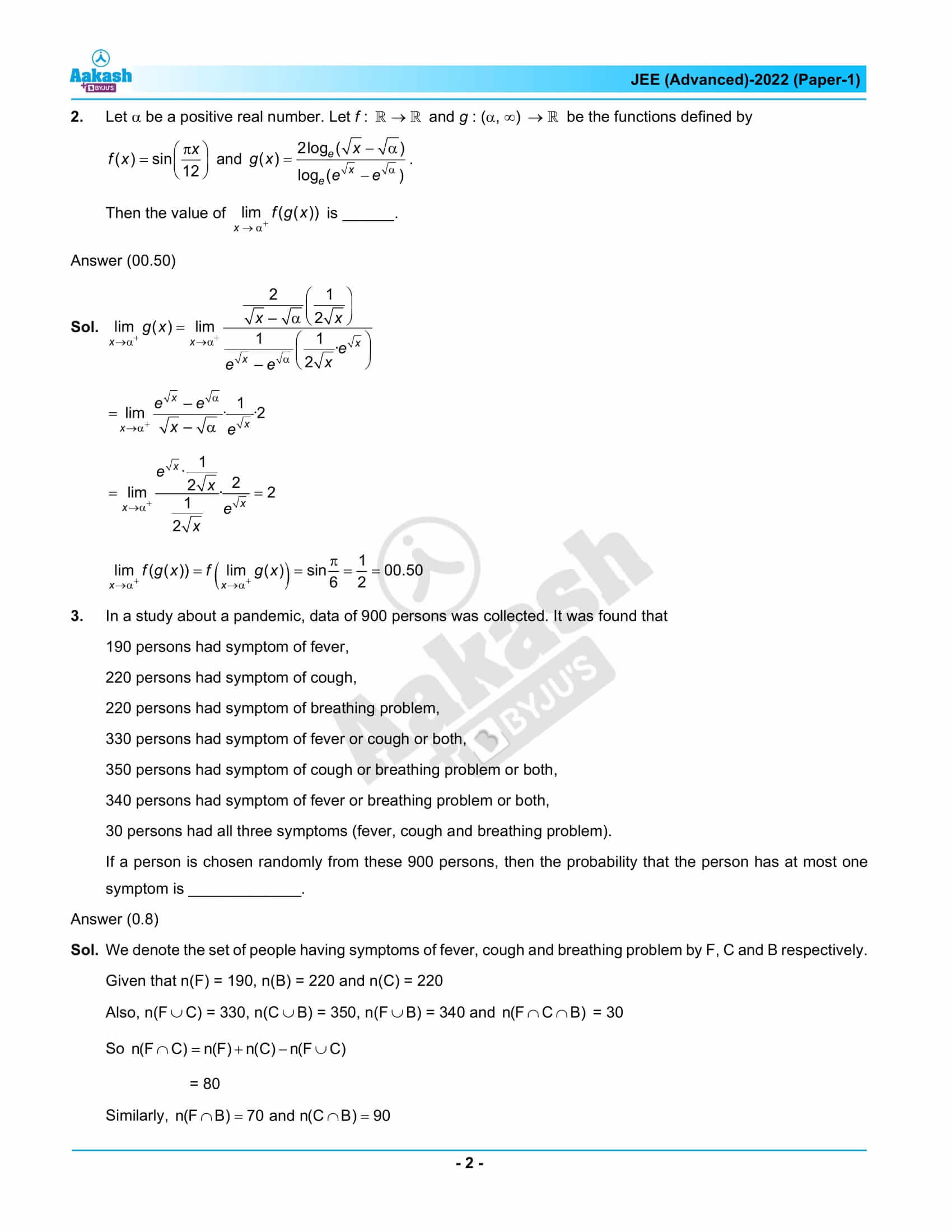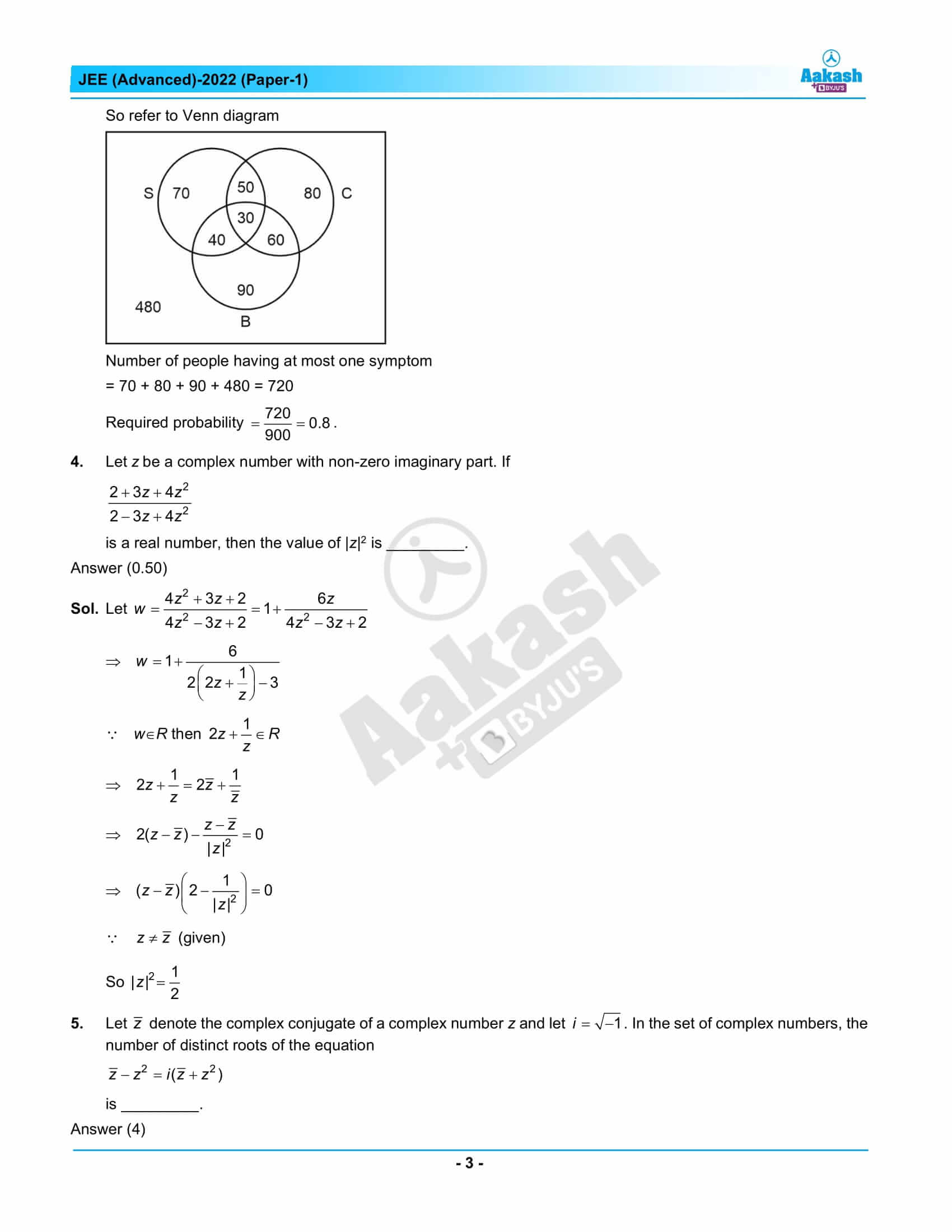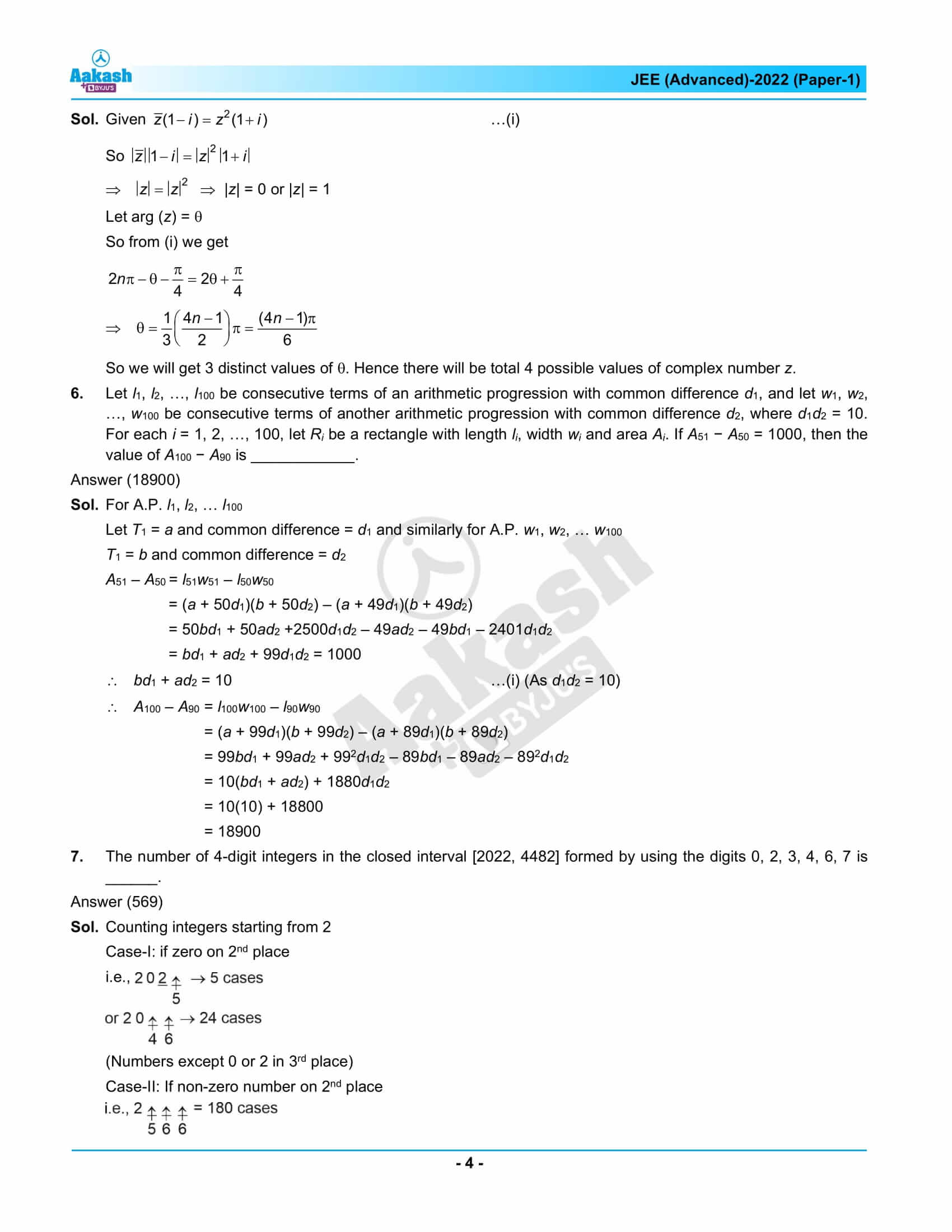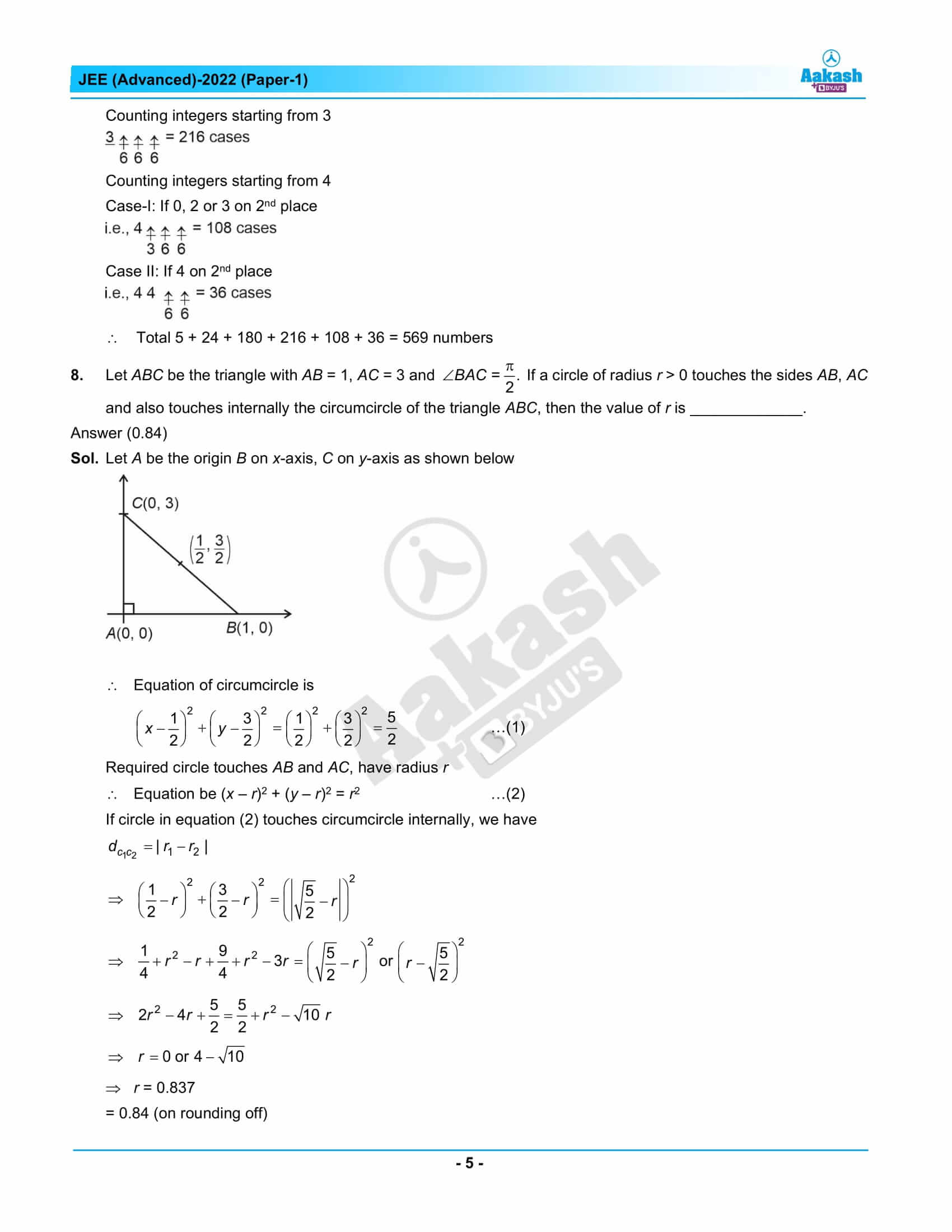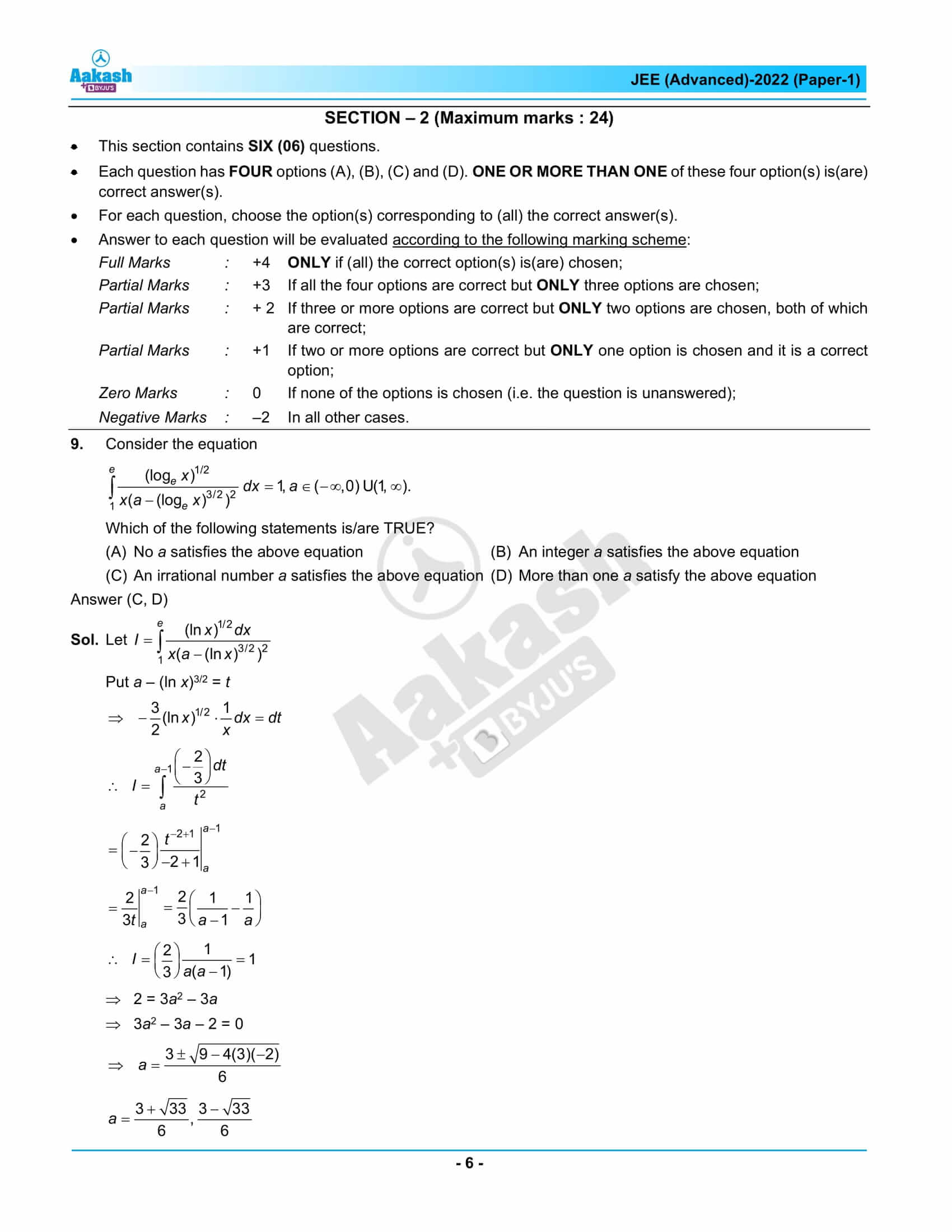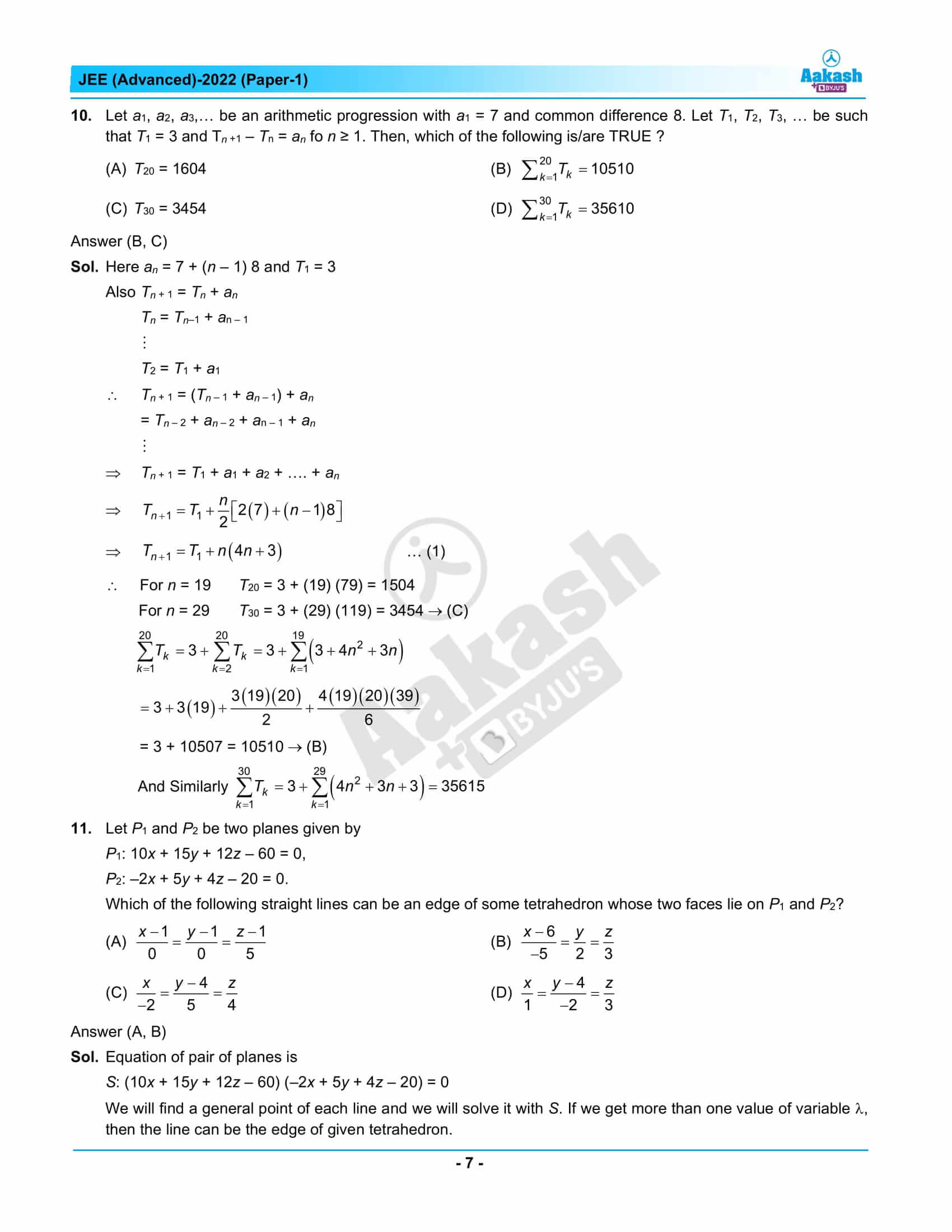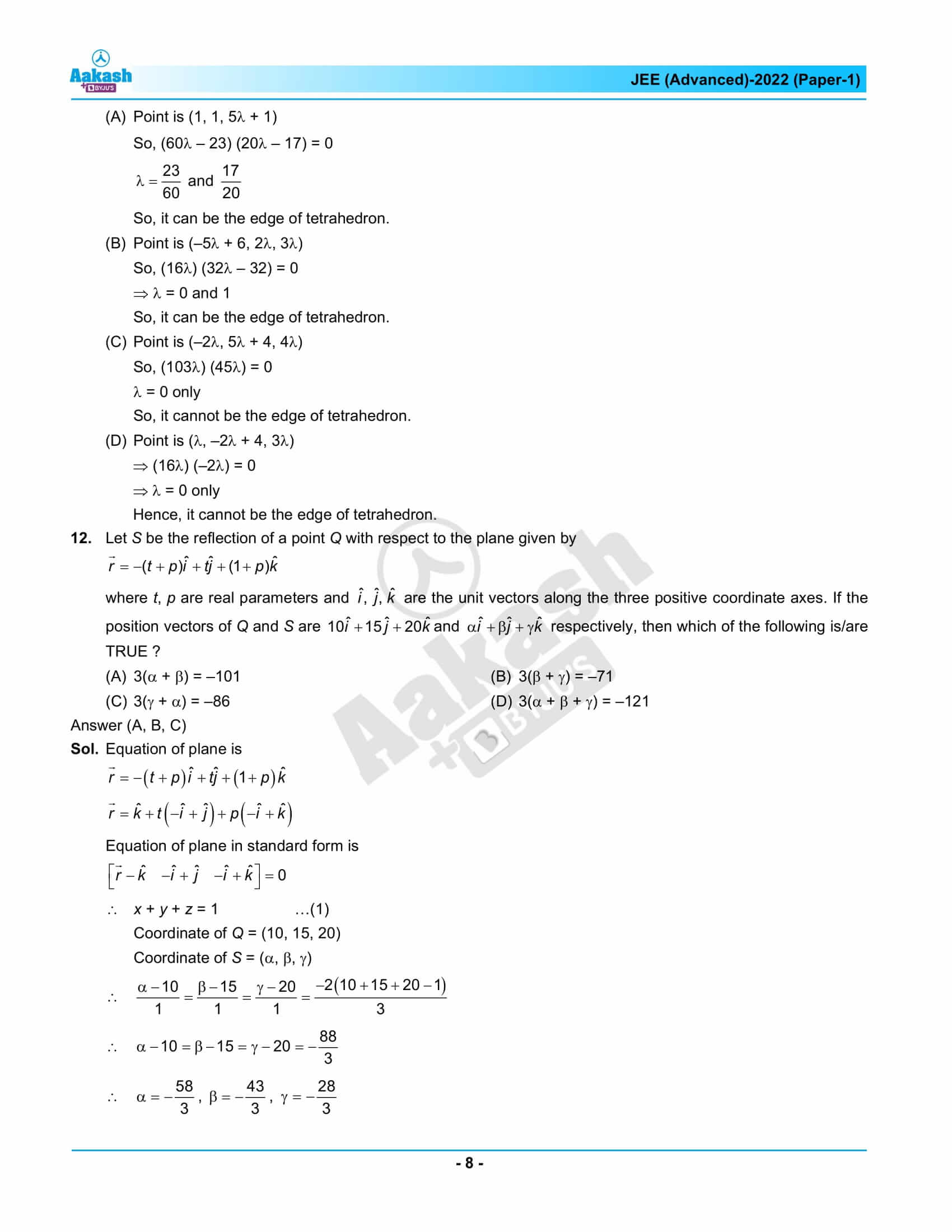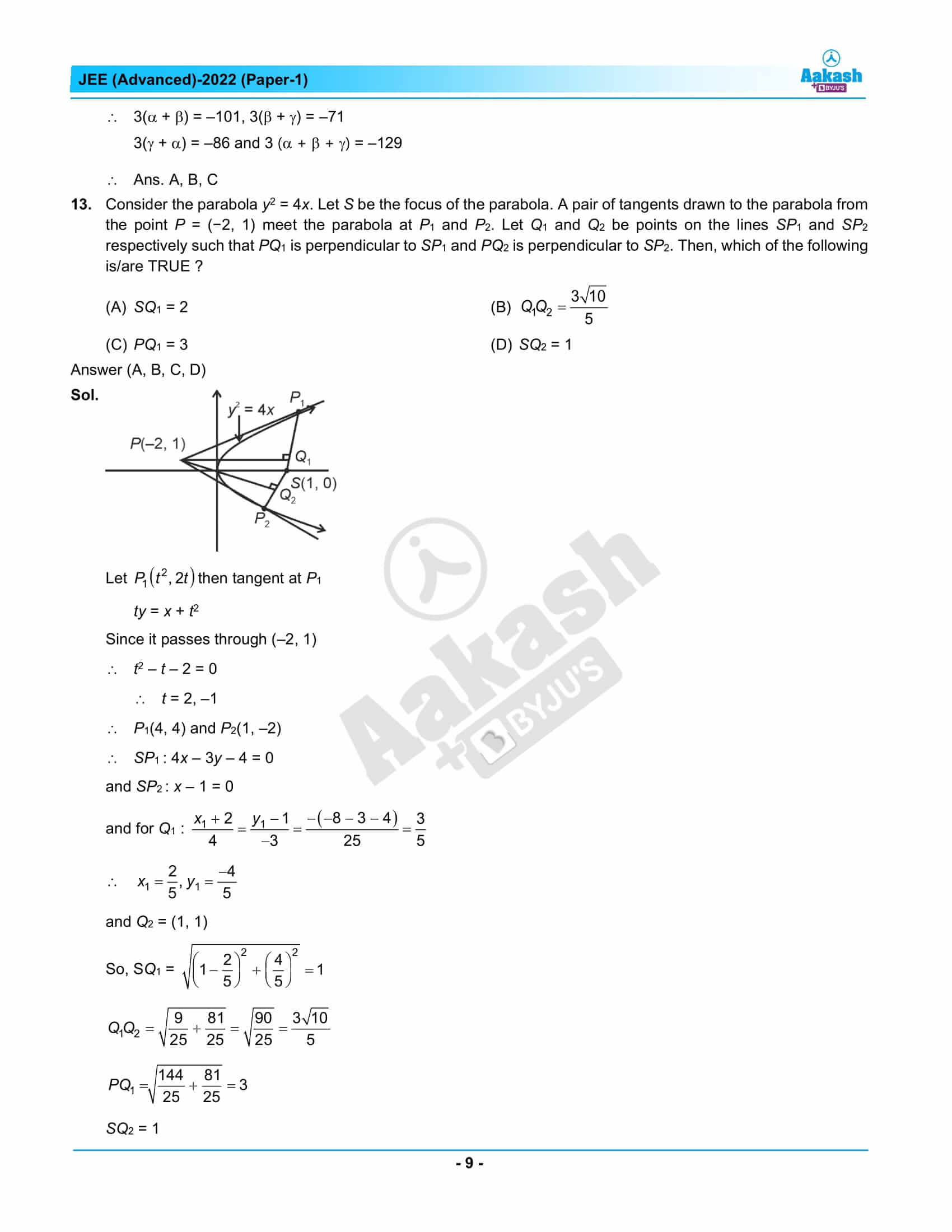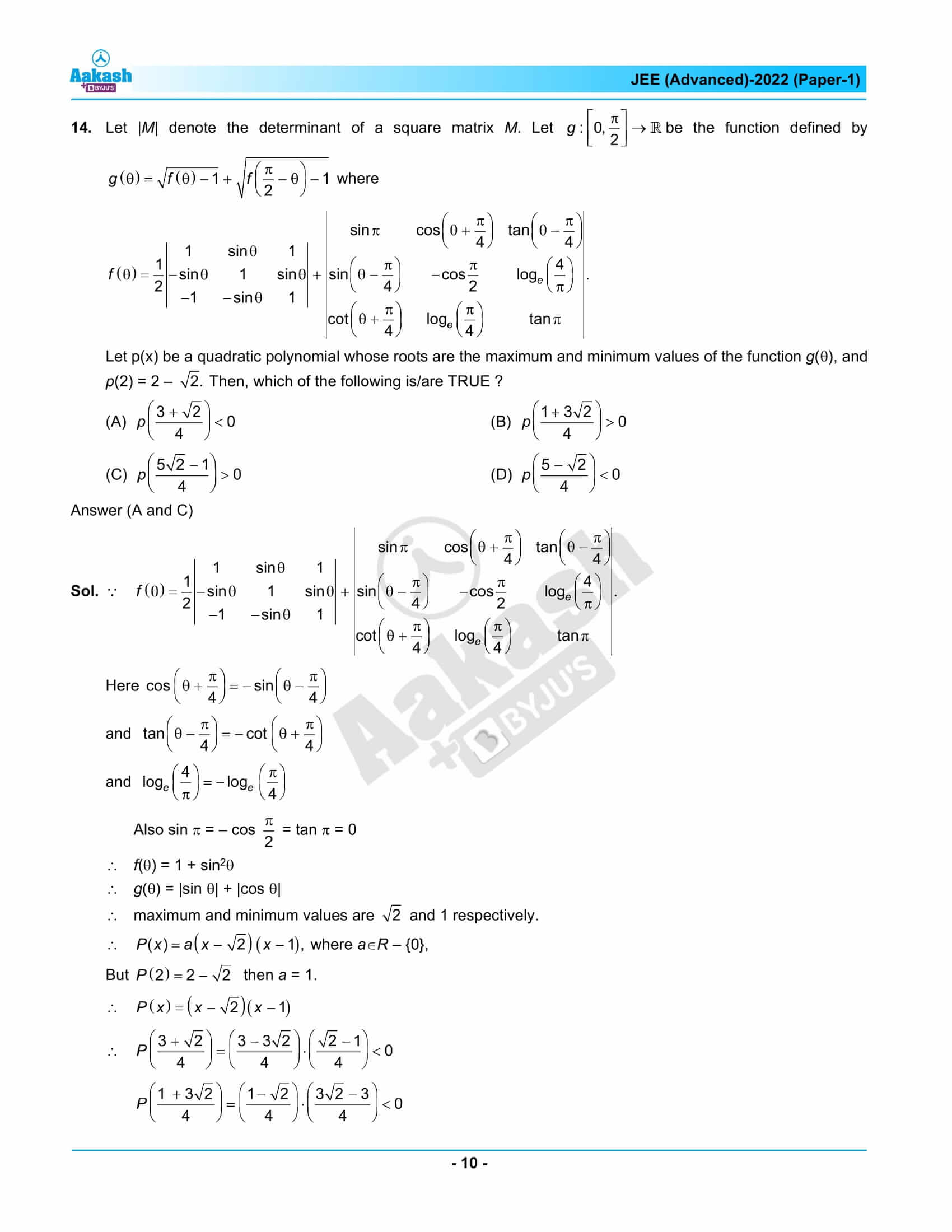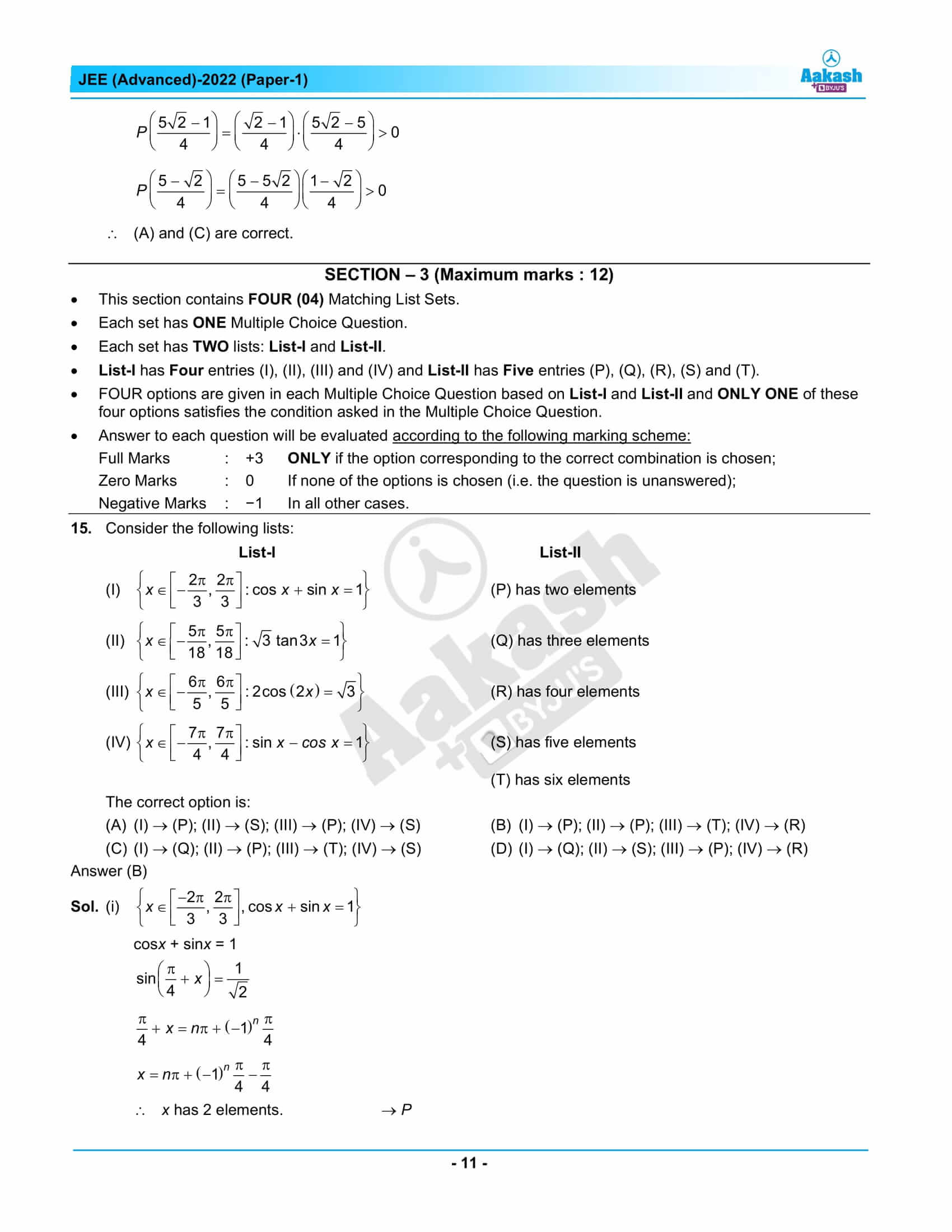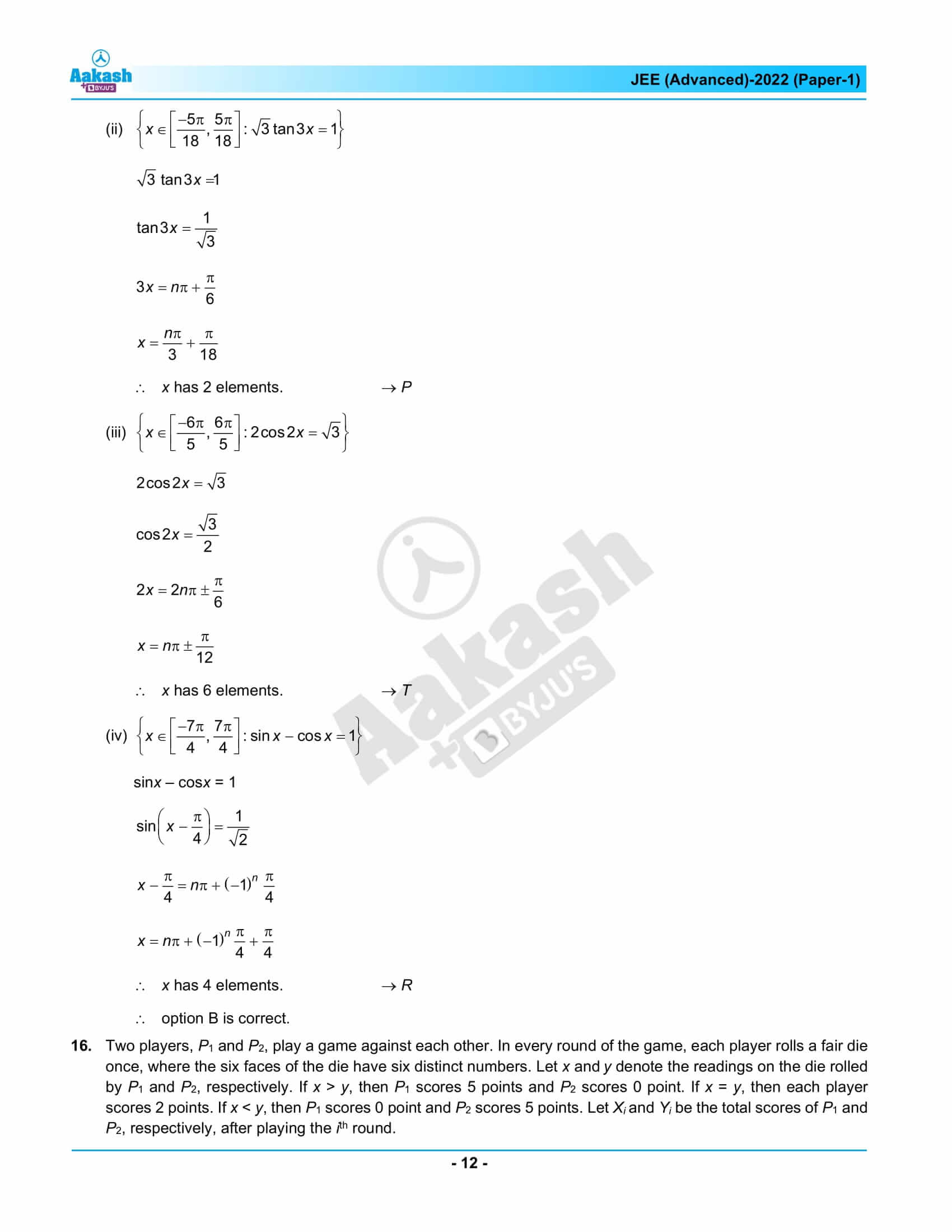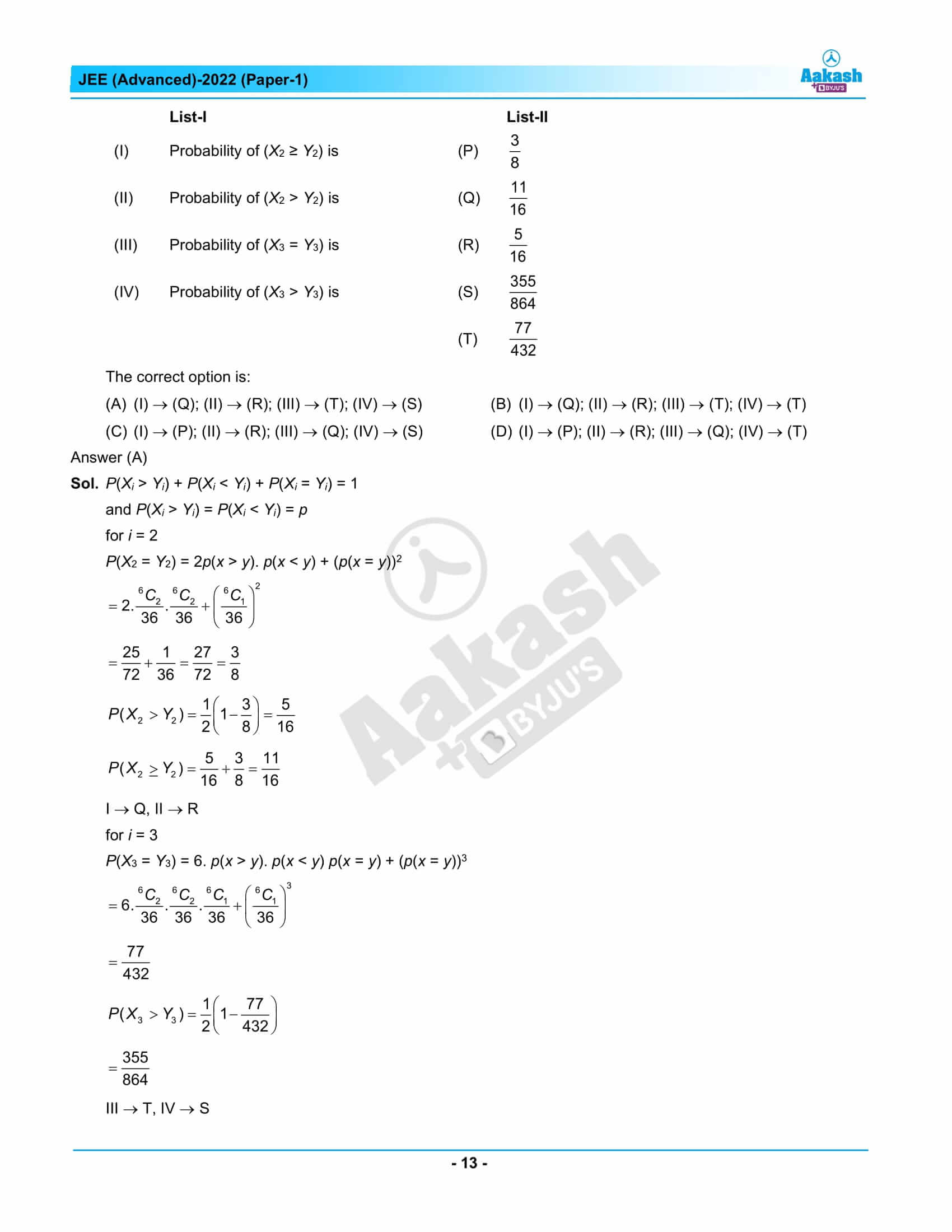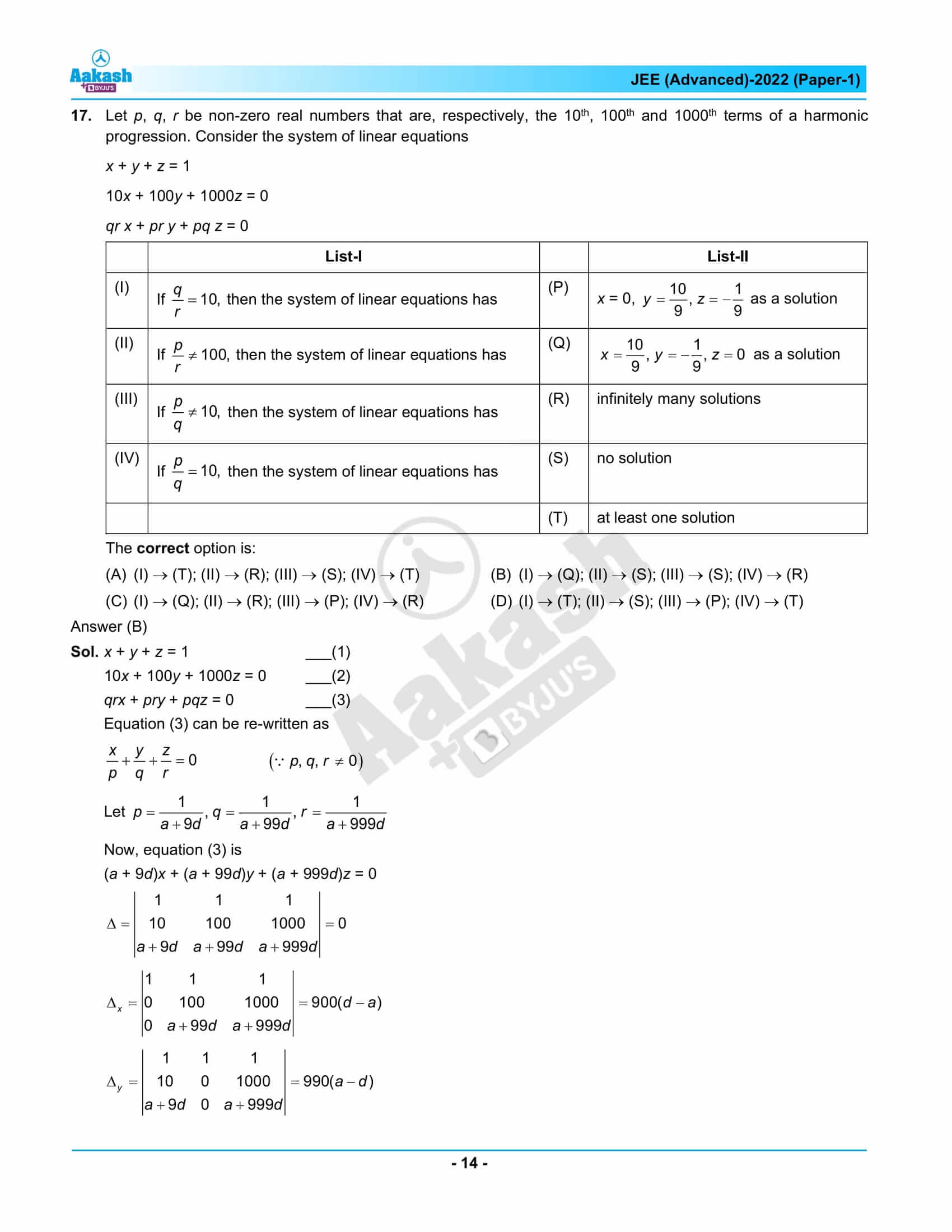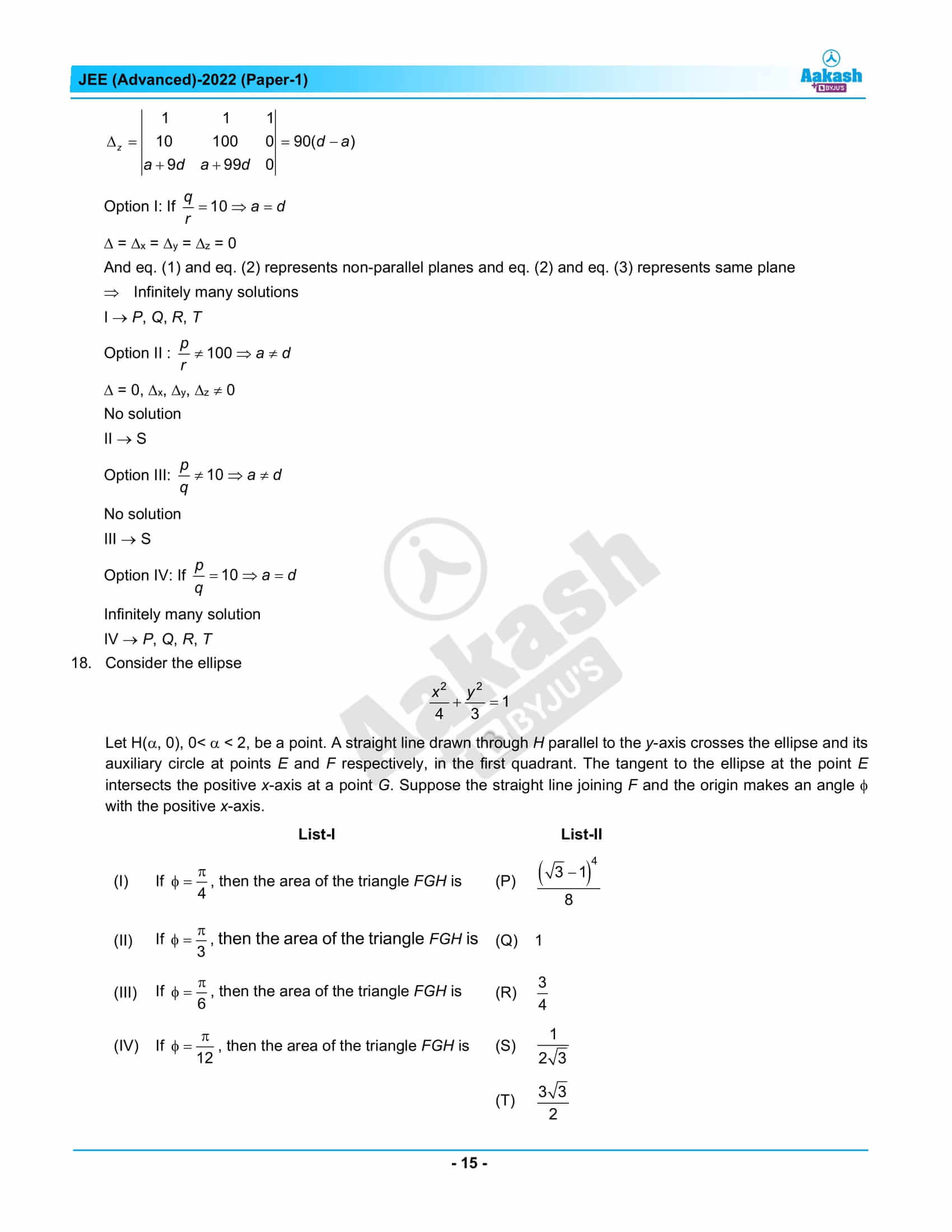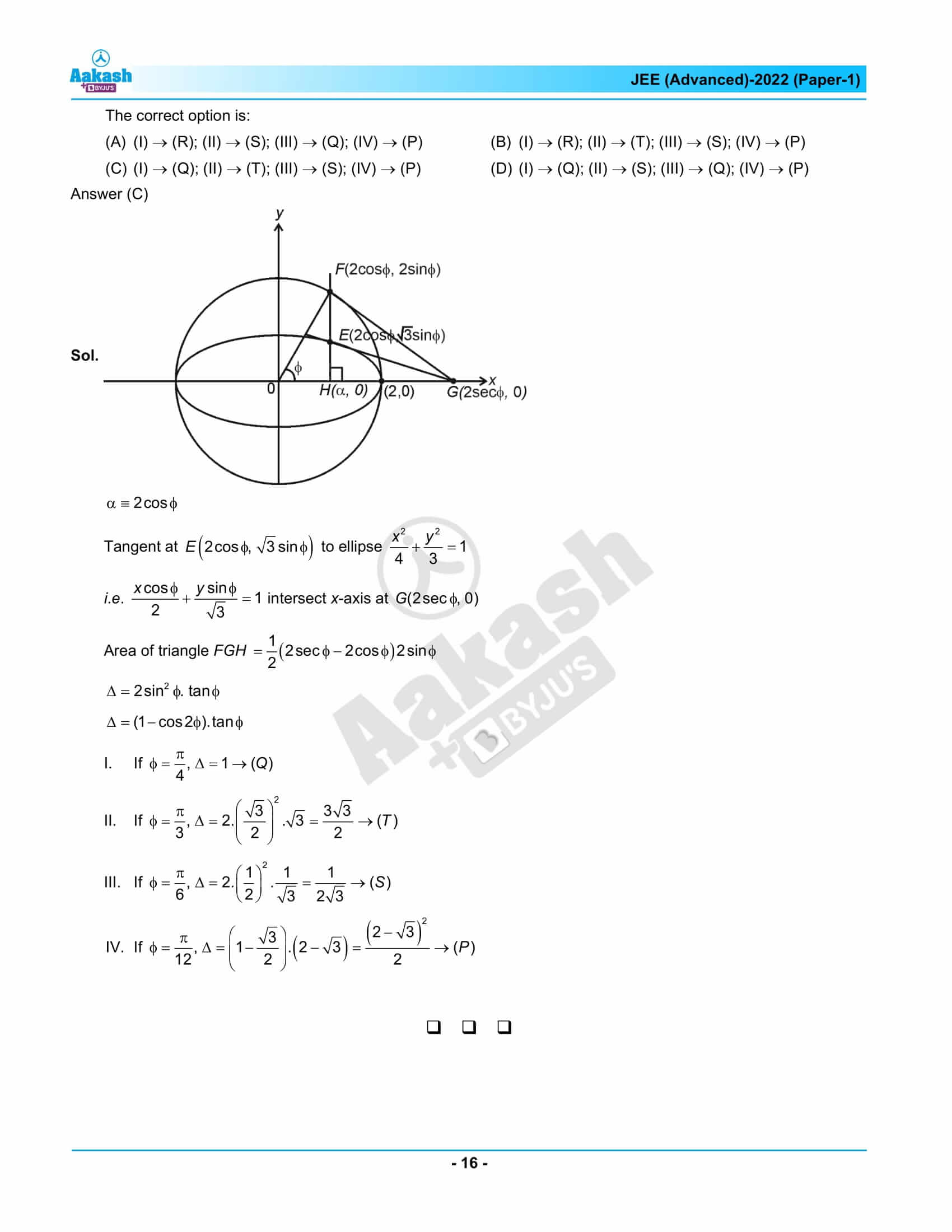## JEE Advanced 2022 Question Paper 1 – Live Paper Analysis and Solutions## Frequently Asked Questions – FAQs

Q1

### How many questions were asked from class 12 in JEE Advanced question paper 2022 Maths paper 1?

8 questions were asked from class 12 for the Maths paper 1 of JEE Advanced question paper 2022.
Q2

### What was the difficulty level of the JEE Advanced 2022 Maths paper 1?

IIT Bombay is well known for setting tough JEE Advanced papers. Thus, JEE Advanced question paper 2022 Maths paper 1 had moderate to difficult questions.
Q3

### How many difficult questions were asked in the JEE Advanced 2022 Maths question paper 1?

3 difficult questions were asked in the JEE Advanced 2022 Maths question paper 1.
Q4

### Which chapter(s) had the easiest question(s) in the JEE Advanced 2022 Maths question paper 1?

An easy question asked from the Trigonometry chapter in the JEE Advanced 2022 Maths question paper 1.
Q5